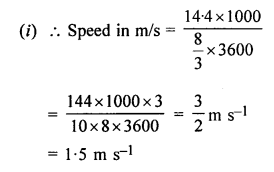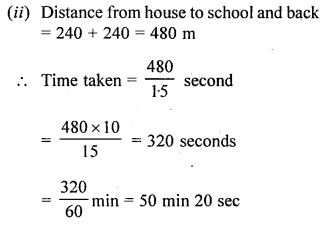## Selina Concise Mathematics Class 6 ICSE Solutions Chapter 17 Idea of Speed, Distance and Time

Selina Publishers Concise Mathematics Class 6 ICSE Solutions Chapter 17 Idea of Speed, Distance and Time

APlusTopper.com provides step by step solutions for Selina Concise ICSE Solutions for Class 6 Mathematics. You can download the Selina Concise Mathematics ICSE Solutions for Class 6 with Free PDF download option. Selina Publishers Concise Mathematics for Class 6 ICSE Solutions all questions are solved and explained by expert mathematic teachers as per ICSE board guidelines.

### Idea of Speed, Distance and Time Exercise 17A – Selina Concise Mathematics Class 6 ICSE Solutions

Question 1.
A train covers 51 km in 3 hours. Calculate its speed. How far does the train go in 30 minutes?
Solution:
Given : Distance = 51 km
Time = 3 hours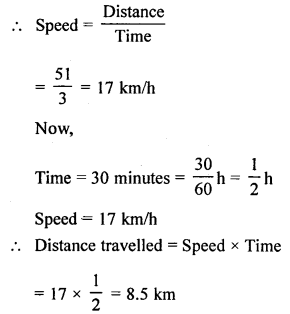Question 2.
A motorist travelled the distance between two towns, which is 65 km, in 2 hours and 10 minutes. Find his speed in metre per minute.
Solution:
Distance between two towns = 65 km
Time taken = 2 hr 10 min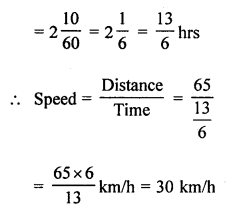Question 3.
A train travels 700 metres in 35 seconds. What is its speed in km/h?
Solution:
Distance = 700 m
Time taken = 35 sec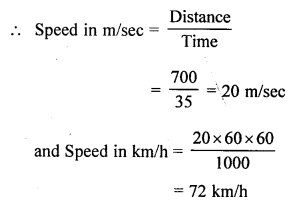Question 4.
A racing car covered 600 km in 3 hours 20 minutes. Find its speed in metre per second. How much distance will the car cover in 50 sec?
Solution:
Distance covered = 600 km
Time taken = 3 hr 20 min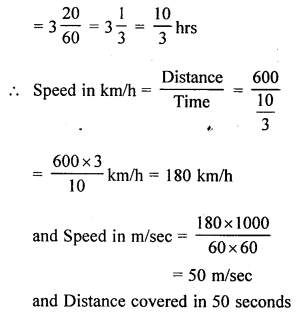= Speed x Time
= 50 x 50 m = 2500 m or 2.50 km

Question 5.
Rohit goes 350 km in 5 hours. Find :
(i) his speed
(ii) the distance covered by Rohit in 6.2 hours
(iii) the time taken by him to cover 210 km.
Solution:
Distance covered = 350 km
Time taken = 5 hours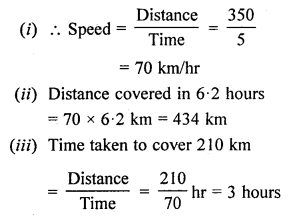Question 6.
A boy drives his scooter with a uniform speed of 45 km/h. Find :
(i) the distance covered by him in 1 hour 20 min.
(ii) the time taken by him to cover 108 km.
(iii) the time taken to cover 900 m.
Solution:
Speed of the scooter = 45 km/h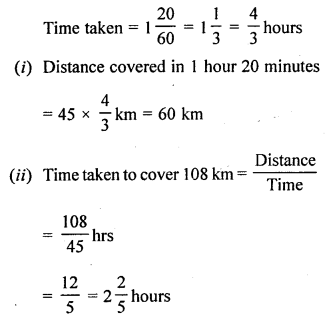Question 7.
I travel a distance of 10 km and come back in 2$$\frac { 1 }{ 2 }$$ hours. What is my speed?
Solution:
Total distance covered = 10 km + 10 km = 20 kmQuestion 8.
A man walks a distance of 5 km in 2 hours. Then he goes in a bus to a nearby town, which is 40 km, in further 2 hours. From there, he goes to his office in an autorickshaw, a distance of 5 km, in $$\frac { 1 }{ 2 }$$ hour. What was his average speed during the whole journey?
Solution:
Distance of 5 km travelled on foot in 2 hours
Distance of 40 km travelled by bus in 2 hours
Distance of 5 km travelled by Rickshaw in $$\frac { 1 }{ 2 }$$ hour
Total distance covered = 5 + 40 + 5 = 50 kmQuestion 9.
Jagan went to another town such that he covered 240 km by a car going at 60 kmh-1. Then he covered 80 km by a train, going at 100 kmh-1 and the rest 200 km, he covered by a bus, going at 50 kmh-1. What was his average speed during the whole journey?
Solution:
Distance covered 240 km by car with speed 60 km/h
Distance covered 80 km by train with speed 100 km/h
and rest distance covered 200 km by bus with speed 50 km/h
Total distance covered = (240 + 80 + 200) km = 520 km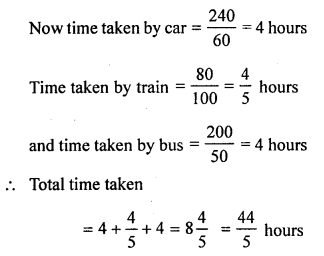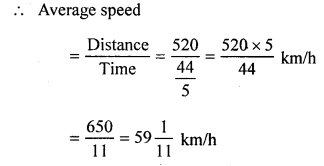Question 10.
The speed of sound in air is about 330 ms-1. Express this speed in kmh-1. How long will the sound take to travel 99 km?
Solution:
Speed of sound in air = 330 m/sec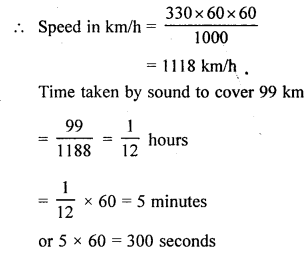### Idea of Speed, Distance and Time Exercise 17B – Selina Concise Mathematics Class 6 ICSE Solutions

Question 1.
A train 180 m long is running at a speed of 90 km/h. How long will it take to pass a railway signal?
Solution:
Distance = 180 m
Speed = 90 km/hQuestion 2.
A train whose length is 150 m, passes a telegraph pole in 10 sec. Find the speed of the train in km/h.
Solution:
Distance = 150 m
Time taken = 10 secQuestion 3.
A train 120 m long passes a railway platform 160 m long in 14 sec. How long will it take to pass another platform which is 100 m long?
Solution:
Distance covered = 120 m + 160 m = 280 m
Time taken = 14 seconds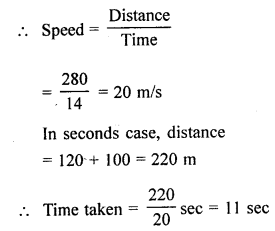Question 4.
Mr. Amit can walk 8 km in 1 hour 20 minutes.
(a) How far does he go in :
(i) 10 minutes ?
(ii) 30 seconds ?
(b) How long will it take him to walk :
(i) 2500 m ?
(ii) 6.5 km ?
Solution:
Amit walks 8 km in 1 hour 20 min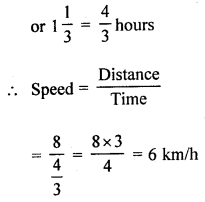Question 5.
Which is greater : a speed of 45 km/h or a speed of 12.25 m/sec?
How much is the distance travelled by each in 2 seconds?
Solution:
First speed = 45 km/h
Second = 12.25 m/sec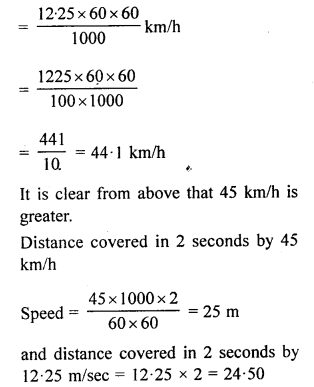Question 6.
A and B start from the same point and at the same time with speeds 15 km/h and 12 km/h respectively, find the distance between A and B after 6 hours if both move in :
(i) same direction
(ii) the opposite directions.
Solution:
A’s speed = 15 km/h
B’s speed = 12 km/h
Distance covered by A in 6 hours = 15 x 6 = 90 km
and Distance covered by B in 6 hours = 12 x 6 = 72 km
(i) Distance between A and B when they move in the same direction = 90 – 72 = 18 km
(ii) Distance between A and B, when they move in the opposite directions = 90 + 72 = 162 km

Question 7.
A and B start from the same place, in the same direction and at the same time with speeds 6 km/h and 2 m/sec respectively. After 5 hours who will be ahead and by how much?
Solution:
A’s speed = 6 km/h
B’s speed = 2 m/sec
Distance covered by A in 5 hours = 6 x 5 = 30 km
and distance covered by B in 5 hours = 5 x 60 x 60 x 2 m = 36000 m
= $$\frac { 3600 }{ 1000 }$$ = 36 km
B will be ahead and 36 – 30 = 6 km ahead.

Question 8.
Mohit covers a certain distance in 6 hrs by his scooter at a speed of 40 kmh-1.
(i) Find the time taken by Manjoor to cover the same distance by his car at the speed of 60 kmh-1.
(ii) Find the speed of Joseph, if he takes 8 hrs to complete the same distance.
Solution:
Mohit’s speed = 40 km/h or kmh-1
Distance covered in = 6 hours
Distance = 40 x 6 = 240 km
(i) Manjoor car’s speed = 60 kmh-1
He will cover the distance of 240 km in = $$\frac { 240 }{ 60 }$$ = 4 hours
(ii) Joseph covered that distance in 8 hours
His speed = $$\frac { 240 }{ 8 }$$ = 30 kmh-1

Question 9.
A boy swims 200 m in still water and then returns back to the point of start in total 10 minutes. Find the speed of his swim in
(i) ms-1
(ii) kmh-1.
Solution:
Distance swimed by a boys of 200 m + 200 m = 400 m
Time taken = 10 minutesQuestion 10.
A distance of 14.4 km is covered in 2 horus 40 minutes. Find the speed in ms-1. With this speed Sakshi goes to her school, 240 m away from her house and then returns back. How much time, in all, will Sakshi take?
Solution:
Distance = 14.4 km
Time taken to cover = 2 hrs 40 min
= $$2\frac { 2 }{ 3 } =\frac { 8 }{ 3 }$$ hrs